Courses

# Test: Biomolecules- 3

## 30 Questions MCQ Test Organic Chemistry | Test: Biomolecules- 3

Description
This mock test of Test: Biomolecules- 3 for Chemistry helps you for every Chemistry entrance exam. This contains 30 Multiple Choice Questions for Chemistry Test: Biomolecules- 3 (mcq) to study with solutions a complete question bank. The solved questions answers in this Test: Biomolecules- 3 quiz give you a good mix of easy questions and tough questions. Chemistry students definitely take this Test: Biomolecules- 3 exercise for a better result in the exam. You can find other Test: Biomolecules- 3 extra questions, long questions & short questions for Chemistry on EduRev as well by searching above.
QUESTION: 1

Solution:
QUESTION: 2

Solution:
QUESTION: 3

### In the reactions,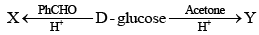The major product X and Y respectively, are:

Solution:
QUESTION: 4

Ident ify the correct set of stereochemical relat ionships amongst the following monosaccharides I-IV: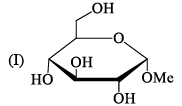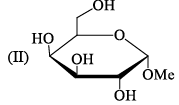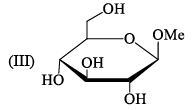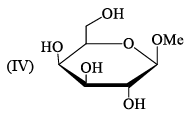Solution:
QUESTION: 5

In a double stranded DNA, if the sequence 5’AGATCC3’ appears on one strand of DNA, what sequence appears on the complementary strand?

Solution:
QUESTION: 6

Which one of the following compounds will form an osazone derivative:

Solution:

When sugars are reacted with excess of phenylhydrazine osazone formation takes place.Osazone will form in that sugar only where keto hydroxy group is present.

Thus CH3CH2COCH2OH will form an osazone derivative; as it has keto hydroxy group.

QUESTION: 7

An acidic amino acid among the following is:

Solution:
QUESTION: 8

Ribose and 2-deoxyribose can be different iated by:

Solution:
QUESTION: 9

The number of disulphide linkage present in insulin are:

Solution:
QUESTION: 10

Which one of the following is an essential amino acid:

Solution:
QUESTION: 11

The pKa values for the three ionizable groups X, Y and Z or Glutamic acid are 4.3, 9.7 and 2.2 respectively: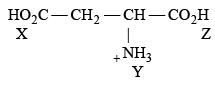The isoelectric point for the amino acid is:

Solution:
QUESTION: 12

The reagent ‘oxine’ commonly used in analytical chemistry is:

Solution:
QUESTION: 13

The structure of D-(+)-glucose is: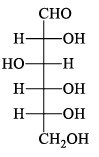The structure of L-(–)-glucose is:

Solution:
QUESTION: 14

Which of the following compounds will behave as a reducing sugar in an aqueous KOH solution:

Solution:
QUESTION: 15

The complementary DNA sequence of the given DNA 5' - G - A - A - T - T - C - 3' is:

Solution:
QUESTION: 16

α-Farnesene shown below is a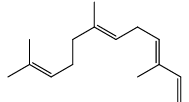Solution:
QUESTION: 17

The correct relationship within each pair of the natural product is

Solution:
QUESTION: 18

Which one among the following is a Sesquiterpene:

Solution:
QUESTION: 19

The compound given below is a: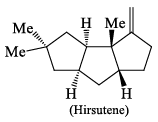Solution:
QUESTION: 20

One sequence of amino acids respects for long distances in silk protein. Complete hydrolysis of one mole of a fragment with this sequence gives 2 mol alanine, 3 mol glycine, and 1 mol serine. Partial hydrolysis yields Ala-Gly-Ala, Gly-Ala-Gly, Gly-Ser-Gly, and Ser-Gly-Ala peptides. What is the amino acid repeat:

Solution:
QUESTION: 21

Tyrosine, at pH 12, has the following structure:

Solution:
QUESTION: 22

A disaccharide does not give a posit ive test for Tollen’s reagent. Upon acidic hydrolysis, it gives an equimolar mixture of two different monosaccharides, both the which can be oxidized by bromine water. This disaccharide is:

Solution:
QUESTION: 23

Match the following compounds with their respective classes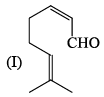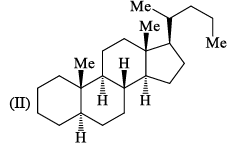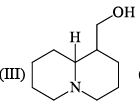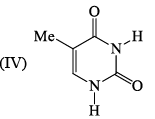Solution:
QUESTION: 24

Predict the products X and Y of the following peptide ligation react ion: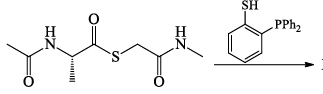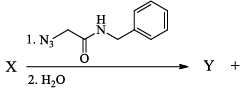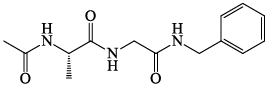Solution:
QUESTION: 25

Which of the following monomers do you expect to find in a RNA molecule:

Solution:
QUESTION: 26

In the following react ion, the major products X and Y are: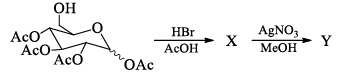Solution:
QUESTION: 27

Among the following, the incorrect statement(s) is/are:

Solution:
QUESTION: 28

All natural amino acids, except one, react with cold nitrous acid and produce nitrogen gas. Which one of the following is that odd amino acid:

Solution:
QUESTION: 29

Which one of the following statement is correct:

Solution:
QUESTION: 30

The product of the following reaction will be able to couple with which amino acids: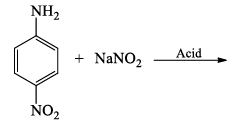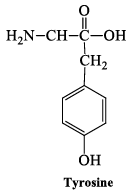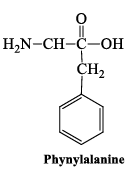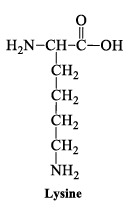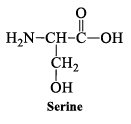Solution: